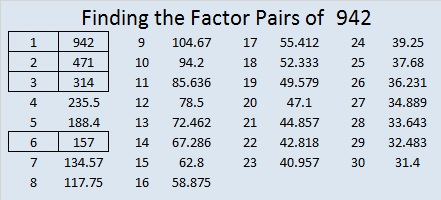# 942 and Level 1

This puzzle is probably as tough as a level 1 puzzle can get, but don’t let that prevent you from giving it a try! Can you figure out where the factors from 1 to 12 go in both the first column and the top row?Print the puzzles or type the solution on this excel file: 12 factors 942-950

Now let me tell you a little about the number 942:

It is the sum of four consecutive prime numbers:
229 + 233 + 239 + 241 = 942

It is the hypotenuse of a Pythagorean triple:
510-792-942 which is 6 times (85-132-157)

942 is a palindrome in two other bases and a repdigit in another:
787 in BASE 11, because 7(11²) + 8(11¹) + 7(11⁰) = 942
272 in BASE 20, because 2(20²) + 7(20¹) + 2(20⁰) = 942
666 in BASE 12, because 6(12²) + 6(12¹) + 6(12⁰) = 6(144+12+1) = 6(157) = 942

942³ is 835,896,888. OEIS.org tells us that 942³ is the smallest perfect cube that contains five 8‘s.

• 942 is a composite number.
• Prime factorization: 942 = 2 × 3 × 157
• The exponents in the prime factorization are 1, 1, and 1. Adding one to each and multiplying we get (1 + 1)(1 + 1)(1 + 1) = 2 × 2 × 2 = 8. Therefore 942 has exactly 8 factors.
• Factors of 942: 1, 2, 3, 6, 157, 314, 471, 942
• Factor pairs: 942 = 1 × 942, 2 × 471, 3 × 314, or 6 × 157
• 942 has no square factors that allow its square root to be simplified. √942 ≈ 30.6920185This site uses Akismet to reduce spam. Learn how your comment data is processed.